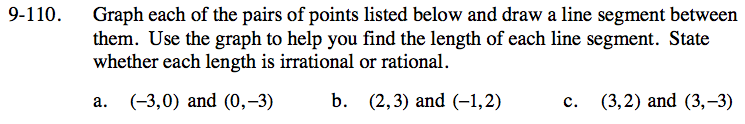### Home > CC3 > Chapter 9 > Lesson 9.2.4 > Problem9-110

9-110.
1. Graph each of the pairs of points listed below and draw a line segment between them. Use the graph to help you find the length of each line segment. State whether each length is irrational or rational. 9-110 HW eTool (Desmos). Homework Help ✎

1. (−3, 0) and (0, −3)

2. (2, 3) and (−1, 2)

3. (3, 2) and (3, −3)Draw a triangle using the segment as your hypotenuse. Then find out the lengths of the legs.

Use the Pythagorean Theorem to find the length of the hypotenuse.

32 + 32 = hypotenuse2
Now solve for the hypotenuse.

$\sqrt{18} \approx 4.24 \;\text{units}$

See part (a).

Use the Pythagrean Theorem to find the length of the segment.

Use the graph to find the length of the segment.

Use the eTool below to graph each segment.
Click the link at right for the full version of the eTool: CC3 9-110 HW eTool